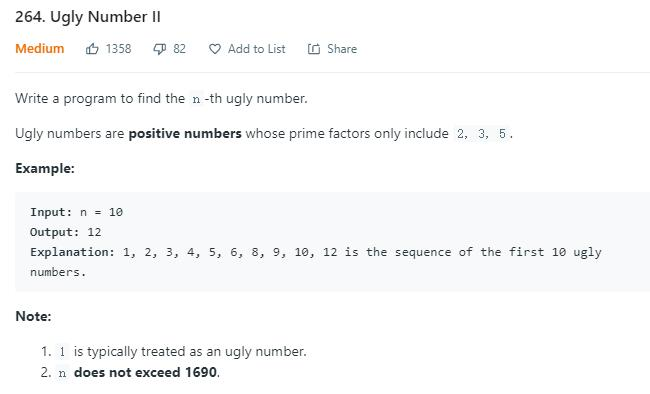# 题目描述（中等难度）# 解法一 暴力

public int nthUglyNumber(int n) {
int count = 0;
int result = 1;
while (count < n) {
if (isUgly(result)) {
count++;
}
result++;
}
//result 多加了 1
return result - 1;
}

public boolean isUgly(int num) {
if (num <= 0) {
return false;
}
while (num % 2 == 0) {
num /= 2;
}
while (num % 3 == 0) {
num /= 3;
}
while (num % 5 == 0) {
num /= 5;
}
return num == 1;
}


public int countPrimes(int n) {
boolean[] notPrime = new boolean[n];
int count = 0;
for (int i = 2; i < n; i++) {
if (!notPrime[i]) {
count++;
//将当前素数的倍数依次标记为非素数
for (int j = 2; j * i < n; j++) {
notPrime[j * i] = true;
}
}
}
return count;
}


public int nthUglyNumber(int n) {
HashSet<Integer> set = new HashSet<>();
int count = 0;
int result = 1;
while (count < n) {
if (set.contains(result)) {
count++;
}
result++;
}
return result - 1;
}


# 解法二

public int nthUglyNumber(int n) {
Queue<Long> queue = new PriorityQueue<Long>();
int count = 0;
long result = 1;
while (count < n) {
result = queue.poll();
// 删除重复的
while (!queue.isEmpty() && result == queue.peek()) {
queue.poll();
}
count++;
queue.offer(result * 2);
queue.offer(result * 3);
queue.offer(result * 5);
}
return (int) result;
}


public int nthUglyNumber(int n) {
TreeSet<Long> set = new TreeSet<Long>();
int count = 0;
long result = 1;
while (count < n) {
result = set.pollFirst();
count++;
}
return (int) result;
}


# 解法三

1, 1×2, 1×3, 2×2, 1×5, 2×3, 2×4, 3×3...

乘 2: 1×2, 2×2, 3×2, 4×2, 5×2, 6×2, 8×2,9×2,…



public int nthUglyNumber(int n) {
int[] ugly = new int[n];
ugly = 1; // 丑数序列
int index2 = 0, index3 = 0, index5 = 0; //三个指针
for (int i = 1; i < n; i++) {
// 三个中选择较小的
int factor2 = 2 * ugly[index2];
int factor3 = 3 * ugly[index3];
int factor5 = 5 * ugly[index5];
int min = Math.min(Math.min(factor2, factor3), factor5);
ugly[i] = min;//更新丑数序列
if (factor2 == min)
index2++;
if (factor3 == min)
index3++;
if (factor5 == min)
index5++;
}
return ugly[n - 1];
}